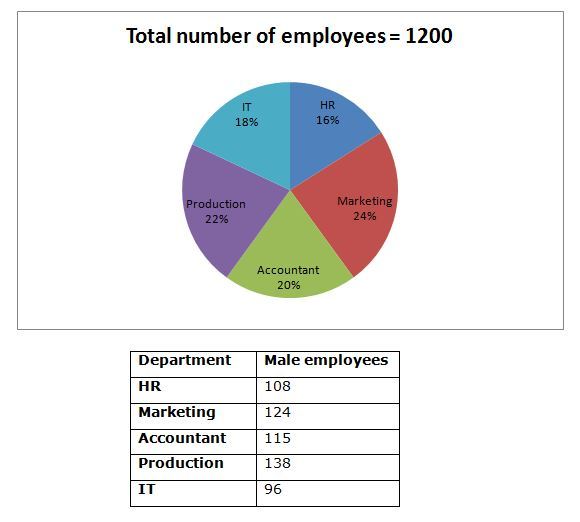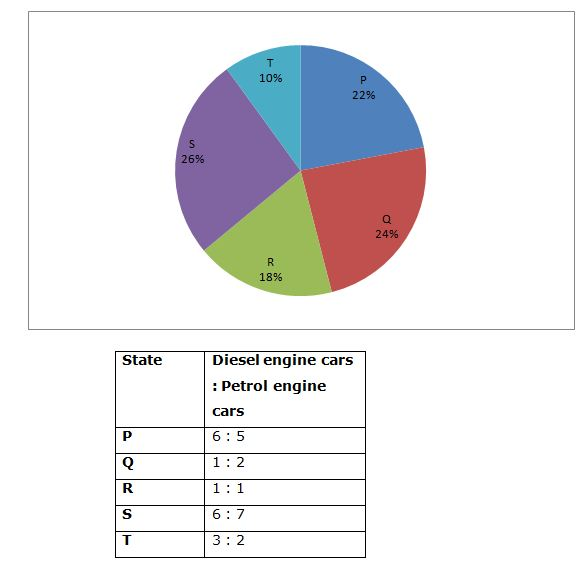# Quantitative Aptitude Questions (Data Interpretation) for SBI Clerk/PO Prelims 2018 Day-140

Dear Readers, SBI is conducting Online Examination for the recruitment of Clerical Cadre and probationary officer. To enrich your preparation here we have providing new series of Data Interpretation – Quantitative Aptitude Questions. Candidates those who are appearing in SBI Clerk/PO Prelims Exams can practice these Quantitative Aptitude average questions daily and make your preparation effective.

[WpProQuiz 2475]

Click “Start Quiz” to attend these Questions and view Solutions

Directions (Q. 1 – 5) Study the following information carefully and answer the given questions:

Following pie chart shows the percentage wise distribution of employees working in various departments and the table shows the total number of male among them.1. Total number of female employees in HR, Accountant and IT department together is approximately what percentage of total number of male employees in the same department together?
1. 118 %
2. 97 %
3. 103 %
4. 88 %
5. 72 %
1. Find the difference between the total number of female employees to that of total number of male employees working all the given department together?
1. 38
2. 52
3. 66
4. 74
5. None of these
1. Total number of employees working in Marketing, Accountant and IT department together is approximately what percentage more/less than the total number of employees working in HR, Accountant and Production department together?
1. 16 % less
2. 7 % more
3. 21 % more
4. 16 % more
5. 7 % less
1. Find the ratio between the total number of male employees working in HR, Accountant and IT department together to that of total number of female employees working in Production, HR and IT department together?
1. 154 : 177
2. 235 : 142
3. 417 : 556
4. 319 : 330
5. None of these
1. Which department has highest number of female employees?
1. Production department
2. Marketing department
3. IT department
4. HR department
5. Accountant department

Directions (Q. 6 – 10) Study the following information carefully and answer the given questions:

Following pie chart shows the percentage wise distribution of cars in 5 different states and the table shows the ratio of diesel engine cars and petrol engine cars.

Total number of cars = 67001. Total number of petrol engine cars in state P, R and T together is what percentage more/less than the total number of diesel engine cars in state Q and S together?
1. 15 % more
2. 24 % less
3. 15 % less
4. 24 % more
5. 30 % less
1. Find the ratio between the total number of cars in state P, Q and R together to that of total number of petrol engine cars in all the given states together?
1. 2235 : 1578
2. 3347 : 2986
3. 3893 : 3052
4. 4288 : 3551
5. None of these
1. Find the total number of diesel engine cars in all the given states together?
1. 2874
2. 3149
3. 3567
4. 2452
5. None of these
1. Find the difference between the total number of petrol engine cars in state P and T together to that of total number of diesel engine cars in state Q and S together?
1. 586
2. 402
3. 448
4. 524
5. None of these
1. Total number of cars in state Q and S together is what percentage of total number of cars in state P and R together?
1. 117 %
2. 132 %
3. 108 %
4. 125 %
5. 96 %

Total number of female employees in HR, Accountant and IT department together

= > [1200*(16/100) – 108] + [1200*(20/100) – 115] + [1200*(18/100) – 96]

= > [192 – 108] + [240 – 115] + [216 – 96]

= > 84 + 125 + 120 = 329

Total number of male employees in HR, Accountant and IT department together

= > 108 + 115 + 96 = 319

Required % = (329/319)*100 = 103.13 % = 103 %

The total number of female employees working all the given department together

= > [1200*(16/100) – 108] + [1200*(24/100) – 124] + [1200*(20/100) – 115] + [1200*(22/100) – 138] + [1200*(18/100) – 96]

= > [192 – 108] + [288 – 124] + [240 – 115] + [264 – 138] + [216 – 96]

= > 84 + 164 + 125 + 126 + 120 = 619

The total number of male employees working all the given department together

= > 108 + 124 + 115 + 138 + 96 = 581

Required difference = 619 – 581 = 38

Total number of employees working in Marketing, Accountant and IT department together

= > 1200*[(24 + 20 + 18)/100] = 1200*[(62)/100]

Total number of employees working in HR, Accountant and Production department together

= > 1200*[(16 + 20 + 22)/100] = 1200*[(58)/100]

Required % = ({1200*[(62)/100] – 1200*[(58)/100]}/ 1200*[(58)/100])*100

= > [(62 – 58)/58]*100 = 6.89 % = 7 % more

The total number of male employees working in HR, Accountant and IT department together

= > 108 + 115 + 96 = 319

The total number of female employees working in Production, HR and IT department together

= > [1200*(16/100) – 108] + [1200*(22/100) – 138] + [1200*(18/100) – 96]

= > [192 – 108] + [264 – 138] + [216 – 96]

= > 84 + 126 + 120 = 330

Required ratio = 319: 330

HR department

= > [1200*(16/100) – 108] = [192 – 108] = 84

Marketing department

= > [1200*(24/100) – 124] = [288 – 124] = 164

Account department

= > [1200*(20/100) – 115] = [240 – 115] = 125

Production department

= > [1200*(22/100) – 138] = [264 – 138] = 126

IT department

= > [1200*(18/100) – 96] = [216 – 96] = 120

Marketing department has highest number of female employees.

Direction (6-10)

Total number of petrol engine cars in state P, R and T together

= > 6700*(22/100)*(5/11) + 6700*(18/100)*(1/2) + 6700*(10/100)*(2/5)

= > 670 + 603 + 268 = 1541

Total number of diesel engine cars in state Q and S together

= > 6700*(24/100)*(1/3) + 6700*(26/100)*(6/13)

= > 536 + 804 = 1340

Required % = [(1541 – 1340)/1340]*100 =15 % more

The total number of cars in state P, Q and R together

= > 6700*(22 + 24 + 18)/100

= > 6700*(64/100) = 4288

The total number of petrol engine cars in all the given states together

= > 6700*(22/100)*(5/11) + 6700*(24/100)*(2/3) + 6700*(18/100)*(1/2) + 6700*(26/100)*(7/13) + 6700*(10/100)*(2/5)

= > 670 + 1072 + 603 + 938 + 268 = 3551

Required ratio = 4288: 3551

The total number of diesel engine cars in all the given states together

= > 6700*(22/100)*(6/11) + 6700*(24/100)*(1/3) + 6700*(18/100)*(1/2) + 6700*(26/100)*(6/13) + 6700*(10/100)*(3/5)

= > 804 + 536 + 603 + 804 + 402

= > 3149

The total number of petrol engine cars in state P and T together

= > 6700*(22/100)*(5/11) + 6700*(10/100)*(2/5)

= > 670 + 268 = 938

The total number of diesel engine cars in state Q and S together

= > 6700*(24/100)*(1/3) + 6700*(26/100)*(6/13)

= > 536 + 804 = 1340

Required difference = 1340 – 938 = 402

Total number of cars in state Q and S together

= > 6700*[(24 + 26)/100]

Total number of cars in state P and R together

= > 6700*[(22+ 18)/100]

Required % = ({6700*[(24 + 26)/100]} / {6700*[(22+ 18)/100]})*100

= > (50/40)*100 = 125 %

Daily Practice Test Schedule | Good Luck

 Topic Daily Publishing Time Daily News Papers & Editorials 8.00 AM Current Affairs Quiz 9.00 AM Quantitative Aptitude “20-20” 11.00 AM Vocabulary (Based on The Hindu) 12.00 PM General Awareness “20-20” 1.00 PM English Language “20-20” 2.00 PM Reasoning Puzzles & Seating 4.00 PM Daily Current Affairs Updates 5.00 PM Data Interpretation / Application Sums (Topic Wise) 6.00 PM Reasoning Ability “20-20” 7.00 PM English Language (New Pattern Questions) 8.00 PM